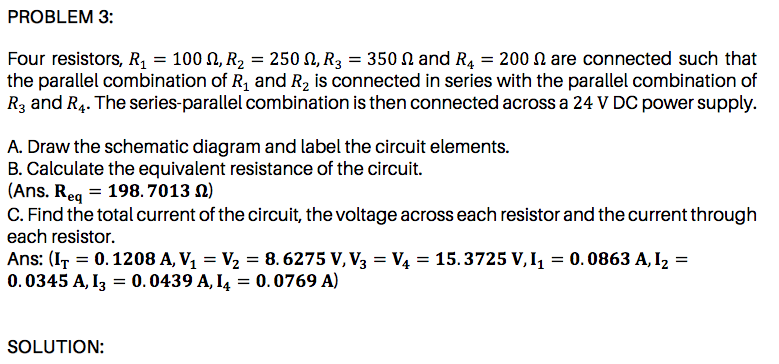# the parallel combination of R, and R2 is connected in series with the parallel combination of R3 and R4. The series-parallel combination is then connected across a 24 V DC power supply. A. Draw the schematic diagram and label the circuit elements. B. Calculate the equivalent resistance of the circuit. |(Ans. Req = 198. 7013 N) C. Find the total current of the circuit, the voltage across each resistor and the current through each resistor.

Questionhelp_outlineImage Transcriptionclosethe parallel combination of R, and R2 is connected in series with the parallel combination of R3 and R4. The series-parallel combination is then connected across a 24 V DC power supply. A. Draw the schematic diagram and label the circuit elements. B. Calculate the equivalent resistance of the circuit. |(Ans. Req = 198. 7013 N) C. Find the total current of the circuit, the voltage across each resistor and the current through each resistor. fullscreen

### Want to see this answer and more?

Experts are waiting 24/7 to provide step-by-step solutions in as fast as 30 minutes!*

*Response times vary by subject and question complexity. Median response time is 34 minutes and may be longer for new subjects.
Tagged in
SciencePhysics

### Current Electricity